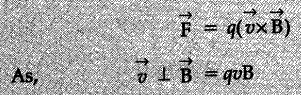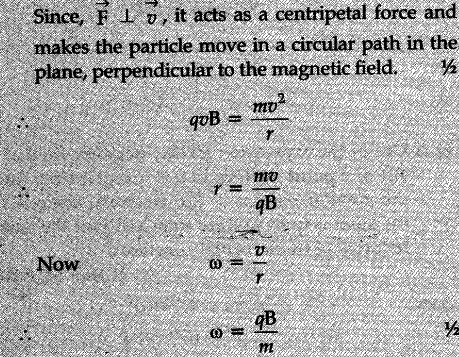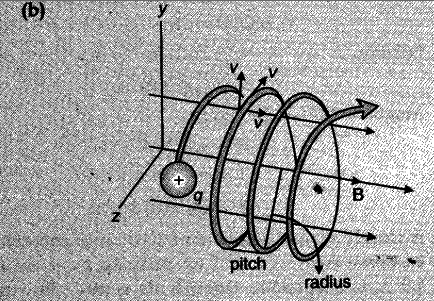# Consider the motion of a charged particle of mass m and charge q moving with velocity v in a magnetic field B

Consider the motion of a charged particle of mass m and charge q moving with velocity v in a
magnetic field B
(a)If v is perpendicular to B, show that its describes a circular path having angular frequency co = qB/m.
(b) If the velocity v has a component parallel to the magnetic field B, trace the path described by the particle. Justify your answer.

(a)Force acting on the charged particle, moving with a velocity v , in a magnetic field B :The component of velocity v parallel to magnetic field, will make the particle move along the field.
The perpendicular component of velocity will cause the particle to move along a circular path in the plane perpendicular to the magnetic field. Hence, the particle will follow a helical path, as shown.